Courses

# Theory of Machines & Vibrations - 1

## 15 Questions MCQ Test GATE Mechanical (ME) 2022 Mock Test Series | Theory of Machines & Vibrations - 1

Description
This mock test of Theory of Machines & Vibrations - 1 for GATE helps you for every GATE entrance exam. This contains 15 Multiple Choice Questions for GATE Theory of Machines & Vibrations - 1 (mcq) to study with solutions a complete question bank. The solved questions answers in this Theory of Machines & Vibrations - 1 quiz give you a good mix of easy questions and tough questions. GATE students definitely take this Theory of Machines & Vibrations - 1 exercise for a better result in the exam. You can find other Theory of Machines & Vibrations - 1 extra questions, long questions & short questions for GATE on EduRev as well by searching above.
QUESTION: 1

Solution:
QUESTION: 2

### A flywheel with a mass of 4 kg has a radius of gyration of 1 m. Find the increase in energy stored in the flywheel when its speed increases from 40 rad/s to 50 rad/s

Solution: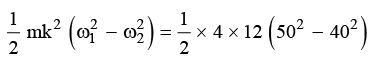= 1800 J

QUESTION: 3

### A crank slotted lever mechanism used in a shaper has a centre distance of 500 mm between the centre of oscillation of the slotted lever and the center of rotation of the crank. The radius of crank is 250 mm. The ratio of cutting stroke to return stroke time is _________.

Solution: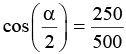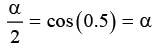= 60 X 2 = 1200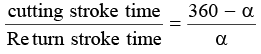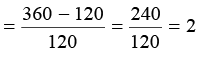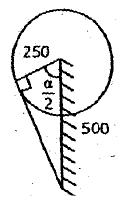QUESTION: 4

A shaft carrying 60 teeth, 1 teeth/mm diameteral pitch gear drives another gear at a speed of 500 rev/min. How fast does the 60 teeth gear rotate if the shaft center distance is 100 mm?

Solution:

Given, T1 = 60

Pi = 1

N2 = 500 & r2 + r2= 100 mm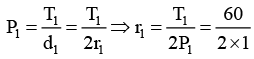= 30 mm

r = 100 − 30= 70 mm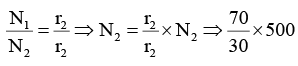= 1167 rev/min

QUESTION: 5

Which of the following mechanism satisfies the four bar linkages shown below?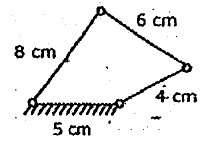Solution:

S + I = 4 + 8 ⇒ 12
P + q = 5 + 6 ⇒ 11
S ÷ I > p + q , therefore if is non-gearshift mechanism to type II mechanisms, are double – rocker

QUESTION: 6

In the linkage shown below, find the number of instantaneous centre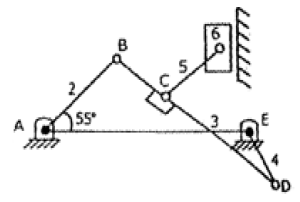Solution: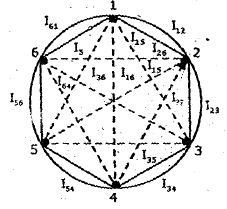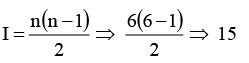QUESTION: 7

The Kutzbach criterion for determining the number of degrees of freedom (n) is (where l = number of links, j = number of joints and h = number of higher pairs)

Solution:
QUESTION: 8

The equation of motion of the washing machine model as shown in the figure is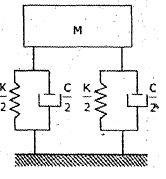Solution:
QUESTION: 9

For high speed engines, the cam follower should move with

Solution:
QUESTION: 10

A cantilever supports a shaft of 100 mm diameter, 1m long which has a mass 500 kg at the end of the shaft with a radius of gyration 450 mm. The frequency of the torsional vibration is _______ Hz. Take rigidity modulus as 80 GN/m2

Solution: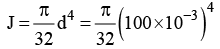= 9.82 ×10−6 m4 ; I = 500 × 0.452
= 101.25kg − m2

Torsional stiffness =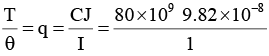= 785.6 ×103 N− m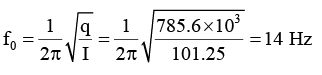QUESTION: 11

Transmission angle is the angle between

Solution:
QUESTION: 12

According to the Kennedy’s theorem, if the three bodies have relative motion among themselves then their instantaneous centers must lie in

Solution:
QUESTION: 13

When the slider moves on a fixed link having a curved surface then the instantaneous centre lies at

Solution:
QUESTION: 14

Six gears are having compound gear train. Gears (2) and (3) are on one shaft. Gear (4) and (5) are on one shaft. The tooth of the Gears 1, 2, 3, 4, 5 and 6 are 30, 60, 40, 20, 30 and 60 respectively. The Train value of the gear train is _________.

Solution:
QUESTION: 15

In Slider-crank mechanism shown in figure, crank O2 A rotates at a constant speed of 1000 rpm in clockwise direction. Determine the acceleration of the crank O2 A when θ2= 45° . Given that the crank length O2A = 6cm & rod length AB = 16cm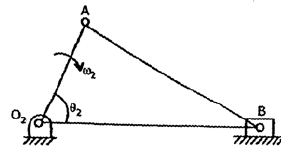Solution: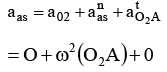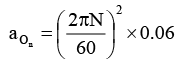= 657.97 = atA = 658 m/s2